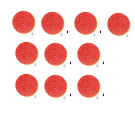Refer to our Texas Go Math Grade 1 Answer Key Pdf to score good marks in the exams. Test yourself by practicing the problems from Texas Go Math Grade 1 Unit 2 Assessment Answer Key.

Vocabulary

Use the words in the box to complete the sentence.

Question 1.
____ to show 6 + 2. (p. 214)

Question 2.
____ to show 9 + 4. (p.244)

Question 3.
___ to show 6 – 2. (p.258)

Concepts and Skills

Useto add and to subtract.

Question 4.
What is 9 – 3? TEKS 1.3.D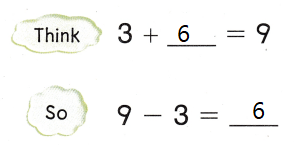Explanation:
The sum of 3 and 6 is 9
The difference of 9 and 3 is 6

Question 5.
What is 10 – 6? TEKS 1.3.D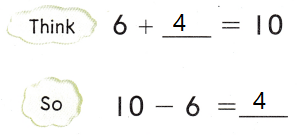Explanation:
The sum of 6 and 4 is 10
The difference of 10 and 6 is 4

Count. Write the total value. TEKS 1.4.A

Question 6.Explanation:
1 dime = 10cents
1 Nickel = 5
1 penny = 1
20 + 5 + 1 = 26cents

Question 7.Explanation:
1 dime = 10 cents
1 nickel = 5 cents
1 penny = 1
10 + 5 + 3 = 18

Texas Test Prep

Question 8.
Which shows a way to take apart 10? TEKS 1.3.D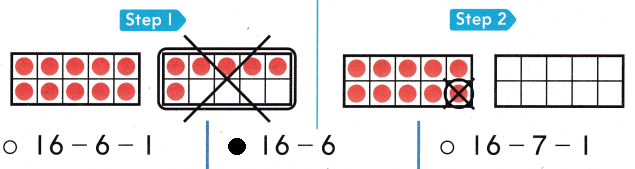Explanation:
16 – 6 = 10
10 is taken apart

Question 9.
Which way shows how to make a ten to solve 9 + 5? TEKS 1.3. D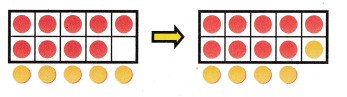(A) 9 + 5 + 4
(B) 9 + 1 + 4
(C) 4 + 5 + 4
Explanation:
9 + 5 = 14
9 + 1 + 4 = 14

Question 10.
Count on to solve 7 + 2. TEKS 1.3.D
(A) 7
(B) 2
(C) 9
Explanation:
7 + 2 = 9
The sum of 7 and 2 is 9.

Question 11.
Which is a doubles fact? TEKS 1.3.D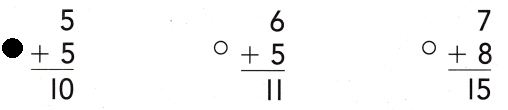Explanation:
5 +5 = 10 is a double fact.

Question 12.
Which coin has a value of 25¢? TEKS 1.4.A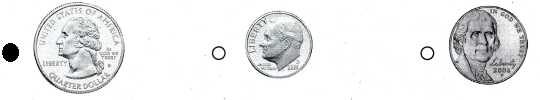Explanation:
25¢ = 1 quarter dollar

Texas Test Prep

Question 13.
Brandy has 2 dimes. Which group of coins has the same value? TEKS 1.4.C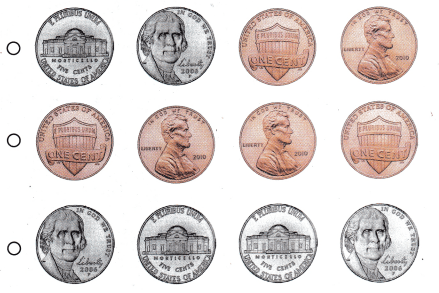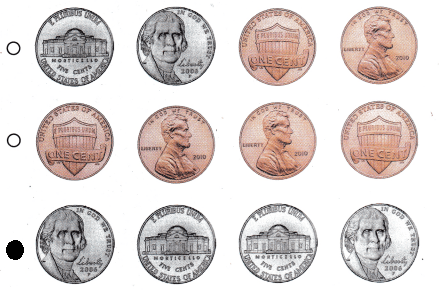Explanation:
1 dime = 10cents
2 x 10 = 20
1 nickel = 5 cents
4 x 5 = 20 cents.

Question 14.
Which shows a way to make a ten to subtract? TEKS 1.3.D
15 – 9 = ___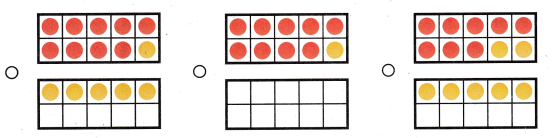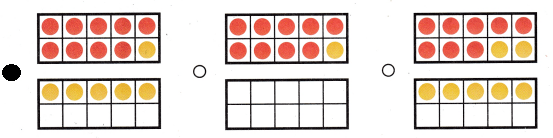Explanation:
15 – 9 = 6
Bubbled the correct option.

Question 15.
Allissa has 12 cupcakes. She needs 15 for a party. How many more cupcakes does she need? TEKS 1.3.E
(A) 10
(B) 8
(C) 3
Explanation:
12 + 3 = 15
She need 3 more cupcakes.

Texas Test Prep

Question 16.
Which subtraction sentence is shown? TEKS 1.3.F(A) 15 – 6 = 9
(B) 11 + 6 = 5
(C) 18 – 8 = 10
Explanation:
18 – 8 = 10
The difference of 18 and 8 is 10.

Question 17.
Useor real objects to solve. Ben chooses 3 addends and finds the sum. He uses the following strategies.

• Make a 10 to add.

Show 3 addends Ben may choose. Write a number sentence and find the sum. Then use pictures or words to describe your model.